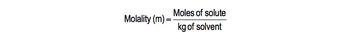## GOB Chemistry

Learn the toughest concepts covered in your GOB - General, Organic, and Biological Chemistry class with step-by-step video tutorials and practice problems.

Solutions

# Molality

Molality (m) represents the amount of solute dissolved per kilogram of solvent.

Understanding Molality
1
example

## Molality2m
Play a video:
morality is depicted as moles of solute per kilograms of solvent morality, which is lower case m. Here we have moles of our salute which is a smaller part of our solution, divided by kilograms of the solvent, the larger portion of our solution. Now this is similar to Mole Arat e, which has capital m. It too, has moles of solute as the numerator. But the denominator is actually leaders of solution. Because of their similarities. There are questions which at times we'll ask you to interchange between the two. Now, in the same way we can expand polarity. The same approach can be applied to morality. So let's say that we have 0.30 mobile of sodium chloride. What does this translate into? Well, this just means that we have 0.30 moles of the Salyut, which is sodium chloride divided by 1 kg of our solvent, which is usually water. Let's say, for example, that we had to five motile off glucose in an acquis solution. Now again, 0.25 model. The number itself means that's how many moles we have off the Salyut. So be 0.25 moles of glucose here we say aqueous solution. Acquis means that are solvent is water, so that would be 1 kg off water. So the take away from this is remember, if they give you morality of a of a compound or solution, it just means that number in terms of moles divided by 1 kg of our solvent. This will be important to remember if we're trying to convert morality to, let's say, mole, Arat e or Mole Fraction or even mass percent. Now that we've learned the basics of morality, click on to the next video and take a look at odds morality.2
concept

## Osmolality56s
Play a video:
Alright, So Ionic Morality or Oz morality represents the morality of dissolved ions within a solution. Now, for example, if we're given 0.30 Molo off sodium chloride, sodium chloride associates into sodium ion and chloride ion, that's a total of two ions involved. If we want the oz morality that's equal to the number of ions, which we said was too times the morality of the compound, which is 0.30 Molo, this will give us an Ionic morality or Oz morality of 60. So remember, when we're talking about Oz morality or ionic morality, we have to take into account the number of ions to disassociate within our given solution. Now that you've seen this, move onto the example left on the bottom of the page and see if your answer matches mine.
3
example

## Calculate Molality Example4m
Play a video:
Ah, solution is prepared by dissolving 43 g of potassium chlorate and enough water to make 100 ml of solution. If the density of the solution is 1.760 g per mil. Leader, what is the morality of potassium chlorate in the solution? We're told the molecular weight of potassium chlorate is 1 22 55 g per mole. All right, so we need to determine the morality. That means we need moles of potassium chlorate divided by kilograms off our solvent. From the given information, we have 43 g of potassium chlorate. We have 100 mls of solution. We have the density of our solution, and we have the molecular weight of potassium chlorate. Now, the molecular way to potassium chlorate can be used to change our grams of potassium chlorate given into moles. So we're gonna stay here for every one mole of potassium chlorate. We're told the masses 1 22 55 Gramps. So grams, cancel out. And now we're gonna have moles. So when we do that, we get 0.350877 moles. So that equals 0.350877 moles here and now we have to determine what our kilograms of solvent are. The only other piece of information that we have left to use is the fact that we have the volume of our solution and the density of our solution. Realize now that we can use these to weaken, multiply them together, to have the volumes cancel out. So when we do that, that gives me 176 g of solution. Now, remember what is in a solution? A solution is made up of Salyut plus solvent together. We already know how many grams of saw you we have so we can subtract it out and realize that when you subtract out the grams of solute, we'll have left our grams of solvent. Here really isn't important what the identity of the solvent is. But it is water because we said that this was in in water. All right, so now we just have to change those 133 g into kilograms. So for every 1 kg, it's 1000 g. So that's 0.133 kg. Take that and plug it here below the moles and we'll have our morality as being two point mol for potassium chlorate solution. So, like we always do, we approach this like a dimensional analysis question. We write down with the information they're asking us to find first. Then we write all the given information from there. We just need to manipulate it and arrange it in a way toe. Isolate the variables that we need, which in this case, was to help us to find morality. So keep this in mind whenever facing any questions, dealing with determining the morality of a solution or an ion.
Molality Calculations
4
example

## Molality Calculations Example 14m
Play a video:
5
example

## Molality Calculations Example 21m
Play a video: